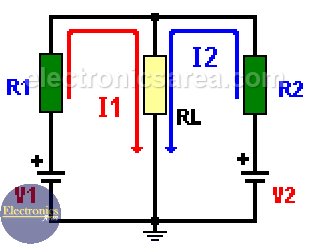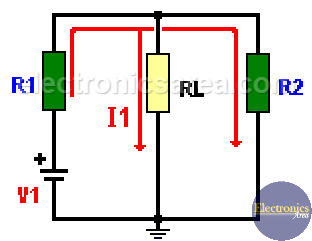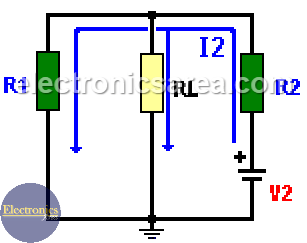# The Superposition Theorem

The Superposition theorem states that the effect of two or more voltage sources in a resistor is equal to the sum of the individual effects of each source taken separately, replacing all the remaining voltage sources with short circuits.

The Superposition theorem is used to find:

• The current values in a circuit with more than one voltage source.
• The voltage values at some point of a circuit that has more than one voltage source.For Example: We want to know what the current through the resistor RL (load) is.

On the original circuit:
R1 = 2 kilohms, R2 = 1 kilohm, RL = 1 kilohm.
V1 = 10 volts, V2 = 20 volts.

Since there are two voltage sources, we use one at a time while shortcutting the other. (In the second diagram we use only V1 and In the third diagram we use only V2). In each case we get the current through the RL resistor. After that, we add the two results to obtain the total current on this resistor.First, we analyze the case where the only voltage source connected is V1.

We get the current delivered by this voltage source obtaining the equivalent resistor of resistors R1 and RL. Req = RL//R2 = 0.5 (kilohms).

Note: // means parallel.

We add R1 to the last result to find the total resistor value (R1 in series with Req.). Then, the total resistance is RT = R1+Req = 0.5+2 = 2.5 kilohms.

This way we found the total equivalent resistor value in series with the voltage source.To obtain the total current we use the Ohm’s Law. I = V/R

Itotal = 10 Volt / 2.5 kilohms = 4 milliamps (mA.)

Using the current division formula we obtain the RL current:
IRL = [IxRL//R2]/RL, where RL//R2 = Req. = 0.5 kilohms)

Replacing: IRL = [4 mA x 0.5 kilohm]/1 kilohm = 2 mA. (milliamps)

The case for the V2 voltage source is developed in the same way. We have to short cut the voltage source V1, and the current through the load resistor (RL) is 8 mA. (milliamps). Adding the two current values we find the total current on the RL resistor of the original circuit

Total RL current: IT = 2 mA + 8 mA = 10 mA (milliamps).

If we know the current (IT) on the RL resistor, we can find the voltage on it using the Ohm’s Law: VL = IT x RL.

•
•
•
•
•
•
•
•
•
•
•
•
•
•
•
•
•
•